### 十个Python实用脚本，快用起来

1、jpg转png

``# 图片格式转换, jpg转png # 方法一 from PIL import Image img = Image.open('test.jpg')img.save('test1.png')  # 方法二 from cv2 import imread, imwrite image = imread("test.jpg", 1)imwrite("test2.png", image)`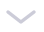`

2、PDF加密和解密

``# PDF加密import pikepdf pdf = pikepdf.open("test.pdf")pdf.save('encrypt.pdf', encryption=pikepdf.Encryption(owner="your_password", user="your_password", R=4))pdf.close()``

``# PDF解密import pikepdf pdf = pikepdf.open("encrypt.pdf",  password='your_password')pdf.save("decrypt.pdf")pdf.close()``

3、获取电脑的配置信息

``# 获取计算机信息import wmi  def System_spec():    Pc = wmi.WMI()    os_info = Pc.Win32_OperatingSystem()    processor = Pc.Win32_Processor()    Gpu = Pc.Win32_VideoController()    os_name = os_info.Name.encode('utf-8').split(b'|')    ram = float(os_info.TotalVisibleMemorySize) / 1048576     print(f'操作系统: {os_name}')    print(f'CPU: {processor.Name}')    print(f'内存: {ram} GB')    print(f'显卡: {Gpu.Name}')     print("\n计算机信息如上 ↑ ↑ ↑ ↑ ↑ ↑ ↑ ↑ ↑ ↑ ↑ ↑")  System_spec()``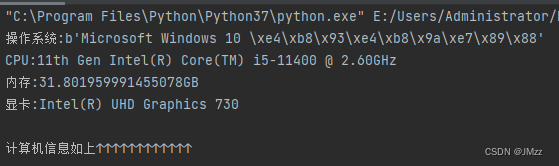4、解压文件

``# 解压文件from zipfile import ZipFile unzip = ZipFile("file.zip", "r")unzip.extractall("output Folder")``

5、Excel工作表合并

6张表，其余表的内容和第一张表都一样。

``import pandas as pd # 文件名filename = "test.xlsx"# 表格数量T_sheets = 5 df = []for i in range(1, T_sheets+1):    sheet_data = pd.read_excel(filename, sheet_name=i, header=None)    df.append(sheet_data) # 合并表格output = "merged.xlsx"df = pd.concat(df)df.to_excel(output)``

6、将图像转换为素描图

``# 图像转换import cv2 # 读取图片img = cv2.imread("img.jpg")# 灰度grey = cv2.cvtColor(img, cv2.COLOR_BGR2GRAY)invert = cv2.bitwise_not(grey)# 高斯滤波blur_img = cv2.GaussianBlur(invert, (7, 7), 0)inverse_blur = cv2.bitwise_not(blur_img)sketch_img = cv2.divide(grey, inverse_blur, scale=256.0)# 保存cv2.imwrite('sketch.jpg', sketch_img)cv2.waitKey(0)cv2.destroyAllWindows()``7、获取CPU温度

``# 获取CPU温度from time import sleepfrom pyspectator.processor import Cpucpu = Cpu(monitoring_latency=1)with cpu:    while True:        print(f'Temp: {cpu.temperature} °C')        sleep(2)``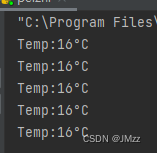8、提取PDF表格

``# 方法一import camelot tables = camelot.read_pdf("tables.pdf")print(tables)tables.export("extracted.csv", f="csv", compress=True) # 方法二, 需要安装Java8import tabula tabula.read_pdf("tables.pdf", pages="all")tabula.convert_into("table.pdf", "output.csv", output_format="csv", pages="all")``

PDF文档的内容如下，包含了一个表格。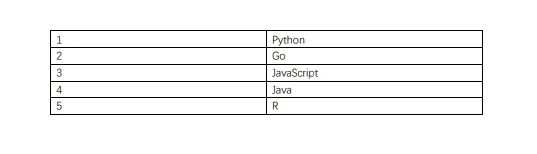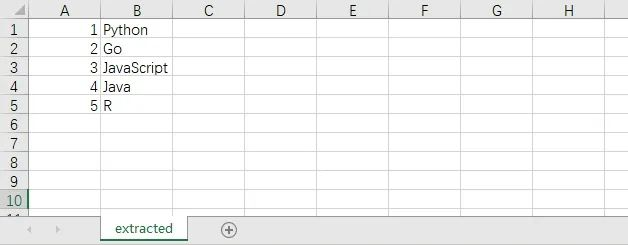9、截图

``# 方法一from mss import msswith mss() as screenshot:    screenshot.shot(output='scr.png') # 方法二import PIL.ImageGrabscr = PIL.ImageGrab.grab()scr.save("scr.png")``

10、拼写检查器

``# 拼写检查# 方法一import textblob text = "meassage"print("original text: " + str(text)) checked = textblob.TextBlob(text)print("corrected text: " + str(checked.correct())) # 方法二import autocorrectspell = autocorrect.Speller(lang='en') # 以英语为例print(spell('cmputr'))print(spell('watr'))print(spell('survice'))``